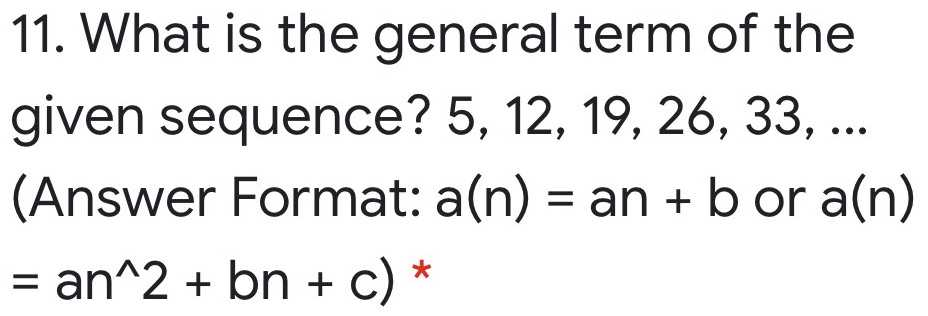### ¿Todavía tienes preguntas de matemáticas?

Pregunte a nuestros tutores expertos
Algebra
Pregunta11. What is the general term of the given sequence? $$5,12,19,26,33 , \ldots$$

(Answer Format: $$a ( n ) = a n + b$$ or $$a ( n )$$$$= a n ^ 2 + b n + c ) ^ { * }$$

$$a(n)= 5+ 7(n- 1)= -2+ 7n$$